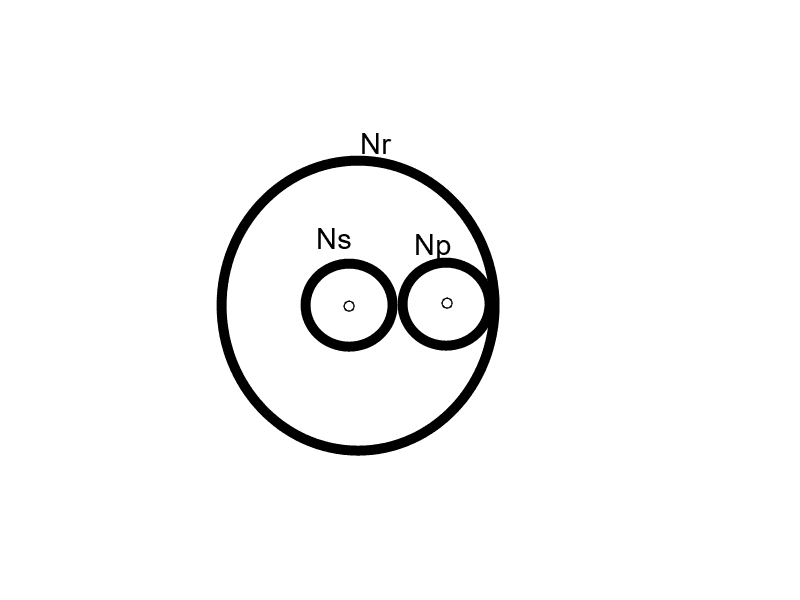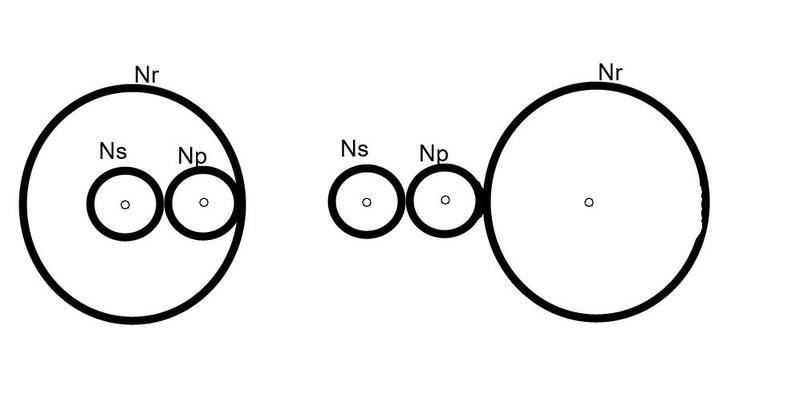# Question about calculation of planetary gear ratios

mrhall1923
So I've found the following formula for calculating the gear ratio of a planetary gear.

Tr Turns of the ring gear
Ts Turns of the sun gear
Ty Turns of the planetary gear carrier
R Ring gear teeth
S Sun gear teeth
P Planet gear teeth
( R + S ) ×Ty = R × Tr + Ts × S

The example I saw on the same website used a fixed ring gear so Tr=0 and the formula worked great.

My question is about having a fixed planetary gear carrier (Ty) with the ring gear being the input and the sun gear being the output. If Ty=0 in the above formula, then the left side of that equation will always be 0 and I'm at a loss at how to solve for Ts.

I have a feeling that there is a better formula for solving this. Can someone help?

Next,

I'm trying to design a 2 stage planetary gearbox with the following.

First planetary gear:
Planetary gear carrier = input
Ring gear = fixed
Sun gear = output (to second planetary gear)

Second planetary gear:
Planetary gear carrier = fixed
Ring gear = input (from first planetary gear)
Sun gear = output

Can anyone provide a single formula for solving the overall gear ratio? So one turn of the first planetary gear carrier will provide how many turns of the second sun gear? I'm looking for a formula that I can use for different size gears of varying tooth quantities.

## Answers and Replies

Machine design texts frequently provide formulas of the sort you are looking to find. You can also work it out for yourself simply by writing the relevant kinematic constraint equations.

CWatters
Science Advisor
Homework Helper
Gold Member
My question is about having a fixed planetary gear carrier (Ty) with the ring gear being the input and the sun gear being the output.

I think this is correct..

Let Nr, Np, Ns be the number of teeth on the ring, planet and sun gears.

For each turn of the ring the planets rotate (about their fixed centre) Nr/Np times.
For each turn of the planets the sun rotates Np/Ns

So for each turn of the ring the sun turns:

(Nr/Np) * (Np/Ns) = Nr/NsEdit: In case it's not obvious, these two set ups are equivalent (except for the direction of rotation).Last edited: